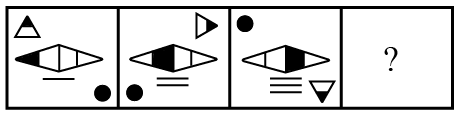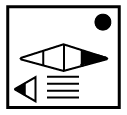Question 6

# Select the figure that can replace the question mark (?) in the following series.Solution

In the given series, the number of vertical lines is increasing by 1 and the triangle is moving one place along the corner of the square and also rotating about itself.

In the next figure, the number of vertical lines will be four and the triangle will be at the bottom left facing left.

$$\therefore\$$The next figure in the series isHence, the correct answer is Option A